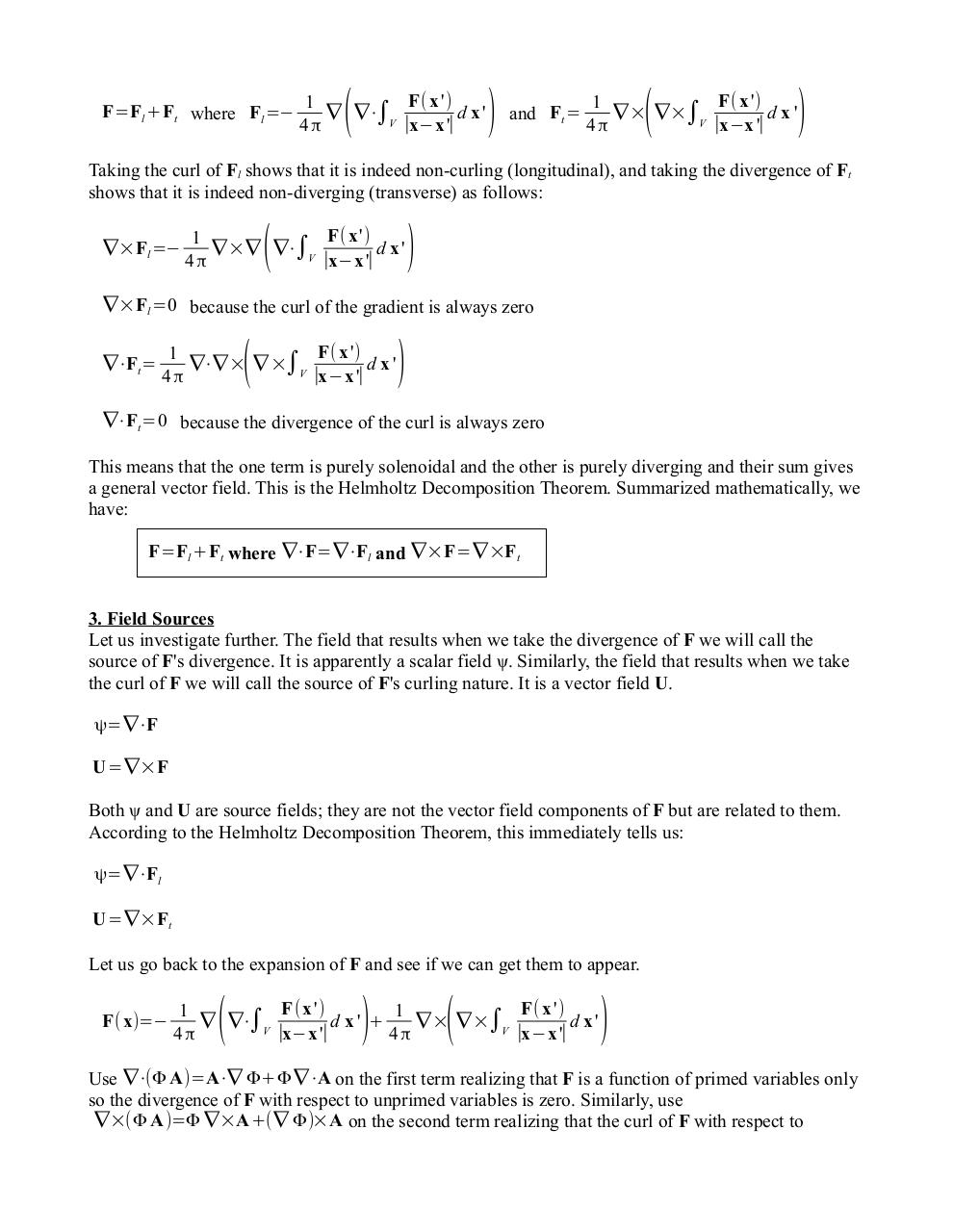# SuppHelmholtzDecomposition.pdfPage 1 2 3 4 5 6

#### Text preview

F=Fl +Ft where Fl =−

(

F(x ')
1
∇ ∇⋅∫V
d x'

x−x '∣

)

and Ft =

(

F( x ')
1
∇ × ∇×∫V
d x'

x−x '∣

)

Taking the curl of Fl shows that it is indeed non-curling (longitudinal), and taking the divergence of Ft
shows that it is indeed non-diverging (transverse) as follows:
∇×Fl =−

(

F( x ')
1
∇ ×∇ ∇⋅∫V
d x'
∣x−x '∣

)

∇×Fl =0 because the curl of the gradient is always zero
∇⋅Ft=

(

F(x ')
1
∇⋅∇ × ∇ ×∫V
d x'
∣x−x '∣

)

∇⋅Ft=0 because the divergence of the curl is always zero

This means that the one term is purely solenoidal and the other is purely diverging and their sum gives
a general vector field. This is the Helmholtz Decomposition Theorem. Summarized mathematically, we
have:
F=Fl +Ft where ∇⋅F=∇⋅Fl and ∇×F=∇ ×Ft
3. Field Sources
Let us investigate further. The field that results when we take the divergence of F we will call the
source of F's divergence. It is apparently a scalar field ψ. Similarly, the field that results when we take
the curl of F we will call the source of F's curling nature. It is a vector field U.
ψ=∇⋅F
U=∇×F

Both ψ and U are source fields; they are not the vector field components of F but are related to them.
According to the Helmholtz Decomposition Theorem, this immediately tells us:
ψ=∇⋅Fl
U=∇×Ft

Let us go back to the expansion of F and see if we can get them to appear.
F( x)=−

(

)

(

F( x ')
F( x ')
1
1
∇ ∇⋅∫V
d x' +
∇ × ∇ ×∫V
d x'

x−x '

x−x '∣

)

Use ∇⋅(Φ A)=A⋅∇ Φ+Φ ∇⋅A on the first term realizing that F is a function of primed variables only
so the divergence of F with respect to unprimed variables is zero. Similarly, use
∇×(Φ A)=Φ ∇×A+(∇ Φ)×A on the second term realizing that the curl of F with respect to